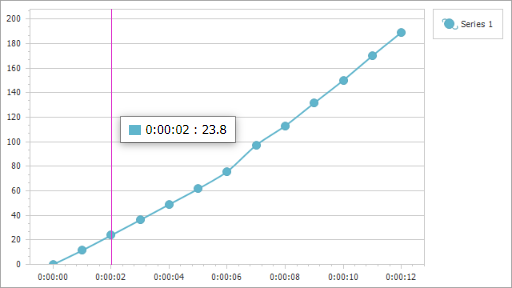# WebChartControl.CalculatedFields Property

Returns a collection of calculated fields.

Namespace: DevExpress.XtraCharts.Web

Assembly: DevExpress.XtraCharts.v22.1.Web.dll

## Declaration

``public ChartCalculatedFieldCollection CalculatedFields { get; }``

## Property Value

Type Description
ChartCalculatedFieldCollection

A collection that stores calculated fields.

## Remarks

Calculated fields allow you to evaluate values based on an expression and use them as a data source for series arguments, values, tooltips, crosshair labels, and so on.

To add a calculated field to a Chart Control, add a ChartCalculatedField object to the CalculatedFields collection and specify the following parameters:

Optional parameters:

• DataSource - Allows you to specify a custom data source to evaluate the specified expression. The calculated field and series must use the same data source. Series can obtain data from the chart’s data source or can be bound to a separate data source (see the Series.DataSource property). If the calculated field’s DataSource property is not specified, the field uses the corresponding series’s data source.

• DataMember - Allows you to specify the name of the sub-list or table if the data source contains more than one distinct list of data items.

• DisplayName - The calculated field’s display name that appears in the Chart Designer‘s Chart Data tab. If DisplayName is not specified, the Name property value is used instead.

## Example

The following example shows how to create a calculated field and then use this field as a data source for a series. The field’s values are calculated by the following expression: [Time.Seconds] * [Velocity].``````<dx:WebChartControl ID="WebChartControl1" runat="server" CrosshairEnabled="True" Height="360px" Width="640px">
...
<CalculatedFields>
<dx:ChartCalculatedField DisplayName="Displacement (m)"
Expression="[Time.Seconds]*[Velocity]"
FieldType="Double"
Name ="Displacement/>
</CalculatedFields>
<SeriesSerializable>
<dx:Series Name="Series 1"
ArgumentDataMember="Time"